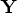Previous Page | Next Page

 Language Reference

## RANKTIE Function

ranks matrix elements by using tie averaging

RANKTIE( matrix)

where matrix is a numeric matrix or literal.

The RANKTIE function creates a new matrix containing elements that are the ranks of the corresponding elements of matrix. The ranks of tied values are averaged.

For example, the following statements produce the vector, as shown:

```
x={2 2 1 0 5};
y=ranktie(x);
```

```
Y

3.5       3.5         2         1         5
```
The RANKTIE function differs from the RANK function in that RANKTIE averages the ranks of tied values, whereas RANK breaks ties arbitrarily.

While the RANK function only ranks the elements of numerical matrices, you can rank the elements of a character matrix by using the UNIQUE function, as demonstrated in the following code:

```
/* Create RANKTIE-like functionality for character matrices */
start ranktiec( x );
s = unique( x );
idx = j(nrow(x), ncol(x));
ctr = 1; /* there can be duplicate values in x */
do i = 1 to ncol( s ); /* for each unique value */
t = loc( x = s[i] );
nDups = ncol( t );
idx[ t ] = ctr+(nDups-1)/2; /* =(ctr:ctr+nDups-1)[:] */
ctr = ctr + nDups;
end;
return ( idx );
finish;

/* call the RANKTIEC module */
x = { every good boy does fine and good and well every day};
rt = ranktiec( x );
print x, rt;
```Previous Page | Next Page | Top of Page